IMO Shortlist 2014 problem G4

Kvaliteta:
Avg: 0,0
Težina:
Avg: 7,0

Consider a fixed circle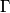$\Gamma$ with three fixed points$A$,$B$ and$C$ on it. Also, let us fix a real number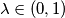$\lambda \in (0, 1)$. For a variable points$P \notin \{A, B, C\}$ on$\Gamma$, let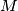$M$ be the point on the segment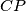$CP$ such that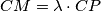$CM = \lambda \cdot CP$. Let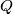$Q$ be the second point of intersection of the circumcircles of the triangles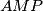$AMP$ and$BMC$. Prove that as$P$ varies, the point$Q$ lies on a fixed circle.

(United Kingdom)

Izvor: https://www.imo-official.org/problems/IMO2014SL.pdf